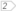#Boost C++ Libraries

...one of the most highly regarded and expertly designed C++ library projects in the world.

This is the documentation for a snapshot of the develop branch, built from commit 446eae3fa0.

## Tutorial

Generating integers in a range
Generating integers with different probabilities
Generating quasi-random line-sphere intersections

### Generating integers in a range

For the source of this example see die.cpp. First we include the headers we need for `mt19937` and `uniform_int_distribution`.

```#include <boost/random/mersenne_twister.hpp>
#include <boost/random/uniform_int_distribution.hpp>
```

We use `mt19937` with the default seed as a source of randomness. The numbers produced will be the same every time the program is run. One common method to change this is to seed with the current time (`std::time(0)` defined in ctime).

```boost::random::mt19937 gen;
```

NoteWe are using a global generator object here. This is important because we don't want to create a new pseudo-random number generator at every call

Now we can define a function that simulates an ordinary six-sided die.

```int roll_die() {boost::random::uniform_int_distribution<> dist(1, 6);return dist(gen);
}
```

`mt19937` produces integers in the range [0, 232-1]. However, we want numbers in the range [1, 6]. The distribution `uniform_int_distribution` performs this transformation.

WarningContrary to common C++ usage `uniform_int_distribution` does not take a half-open range. Instead it takes a closed range. Given the parameters 1 and 6, `uniform_int_distribution` can produce any of the values 1, 2, 3, 4, 5, or 6.

A distribution is a function object. We generate a random number by calling `dist` with the generator.

### Generating integers with different probabilities

For the source of this example see weighted_die.cpp.

```#include <boost/random/mersenne_twister.hpp>
#include <boost/random/discrete_distribution.hpp>

boost::mt19937 gen;
```

This time, instead of a fair die, the probability of rolling a 1 is 50% (!). The other five faces are all equally likely.

`discrete_distribution` works nicely here by allowing us to assign weights to each of the possible outcomes.

TipIf your compiler supports `std::initializer_list`, you can initialize `discrete_distribution` directly with the weights.

```double probabilities[] = {
0.5, 0.1, 0.1, 0.1, 0.1, 0.1
};
boost::random::discrete_distribution<> dist(probabilities);
```

Now define a function that simulates rolling this die.

```int roll_weighted_die() {return dist(gen) + 1;
}
```

 Add 1 to make sure that the result is in the range [1,6] instead of [0,5].

For the source of this example see password.cpp.

This example demonstrates generating a random 8 character password.

```#include <boost/random/random_device.hpp>
#include <boost/random/uniform_int_distribution.hpp>
#include <iostream>

int main() {std::string chars(
"abcdefghijklmnopqrstuvwxyz"
"ABCDEFGHIJKLMNOPQRSTUVWXYZ"
"1234567890"
"!@#\$%^&*()"
"`~-_=+[{]}\\|;:'\",<.>/? ");boost::random::random_device rng;boost::random::uniform_int_distribution<> index_dist(0, chars.size() - 1);
for(int i = 0; i < 8; ++i) {
std::cout << chars[index_dist(rng)];
}
std::cout << std::endl;
}
```

 We first define the characters that we're going to allow. This is pretty much just the characters on a standard keyboard. We use `random_device` as a source of entropy, since we want passwords that are not predictable. Finally we select 8 random characters from the string and print them to cout.

### Generating quasi-random line-sphere intersections

For the source of this example see intersections.cpp.

This example demonstrates generating quasi-randomly distributed chord entry and exit points on an S2 sphere.

First we include the headers we need for `niederreiter_base2` and `uniform_01` distribution.

```#include <boost/random/niederreiter_base2.hpp>
#include <boost/random/uniform_01.hpp>

#include <boost/math/constants/constants.hpp>

#include <boost/tuple/tuple.hpp>
```

We use 4-dimensional `niederreiter_base2` as a source of randomness.

```boost::random::niederreiter_base2 gen(4);

int main()
{
typedef boost::tuple<double, double, double> point_t;

const std::size_t n_points = 100; // we will generate 100 points

std::vector<point_t> points;
points.reserve(n_points);boost::random::uniform_01<double> dist;

for (std::size_t i = 0; i != n_points; ++i)
{
```

Using formula from J. Rovira et al., "Point sampling with uniformly distributed lines", 2005 to compute uniformly distributed chord entry and exit points on the surface of a sphere.

```double cos_theta = 1 - 2 * dist(gen);
double sin_theta = std::sqrt(1 - cos_theta * cos_theta);
double phi = boost::math::constants::two_pi<double>() * dist(gen);
double sin_phi = std::sin(phi), cos_phi = std::cos(phi);

point_t point_on_sphere(sin_theta*sin_phi, cos_theta, sin_theta*cos_phi);
```

Here we assume that our sphere is a unit sphere at origin. If your sphere was different then now would be the time to scale and translate the `point_on_sphere`.

```  points.push_back(point_on_sphere);
}
```

Vector `points` now holds generated 3D points on a sphere.

```  return 0;
}
```

 `niederreiter_base2` produces integers in the range [0, 264-1]. However, we want numbers in the range [0, 1). The distribution `uniform_01` performs this transformation.